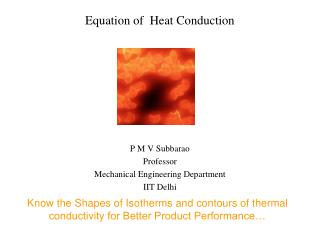DownloadDownload PresentationEquation of Heat Conduction

# Equation of Heat Conduction

Télécharger la présentation## Equation of Heat Conduction

- - - - - - - - - - - - - - - - - - - - - - - - - - - E N D - - - - - - - - - - - - - - - - - - - - - - - - - - -
##### Presentation Transcript

1. Equation of Heat Conduction P M V Subbarao Professor Mechanical Engineering Department IIT Delhi Know the Shapes of Isotherms and contours of thermal conductivity for Better Product Performance…

2. Analysis of Cause Rate of Heat condition in/out Rate of Heat condition in/out Rate of Heat condition in/out Rate of energy generation Rate of Heat condition in/out ?????

3. Apply first law of thermodynamics to the element to get

4. The thermal energy balance equation Using Gauss Divergence Theorem:

5. The thermal energy balance equation for the volume V is then However, since the integrals are equal the arguments are also equal and the most general form of the differential thermal energy balance equation is This is called the differential thermal energy balance equation.

6. Constitutive Equation: The Heat Flux On a principle Plane :

7. Anisotropic thermal conductivity • In quartz, perpendicular to the c-axis, the thermal conductivity is 6.5 Wm-1K-1. (kxx =kyy) • The thermal conductivity parallel to c (kzz) is 11.3 Wm-1K-1.

8. Thin Plate : Anisotropi Materal • Here the geometry of the set-up constrains the temperature gradient to be perpendicular to the plate. • Due to the anisotropic nature of the material, the heat flux, J, will be in the direction shown, say. • However, the thermal conductivity perpendicular to the plate is defined as the component of the heat flux parallel to the temperature gradient, q||, divided by the magnitude of that gradient. • Thus: q

9. Rod : Anisotropic • Now the heat must flow along the rod, and the temperature gradient will be in a different direction, as shown. • It is important to realize that in anisotropic materials

10. The conductivity Tensor Most of the crystalline solids show symmetric behavior. Based on theory of symmetry:

11. Generalized Fourier’s Law of Conduction Most common engineering materials are isotropic in nature.

12. The Conduction Equation Incorporation of the constitutive equation of an isotropic material into the energy equation above yields: Dividing both sides by rCpand introducing the thermal diffusivity of the material given by

13. Thermal Diffusivity • Thermal diffusivity includes the effects of properties like mass density, thermal conductivity and specific heat capacity. • Thermal diffusivity, which is very important in all unsteady heat-conduction problems, is a property of the solid object. • The time rate of change of temperature depends on its numerical value. • The physical significance of thermal diffusivity is associated with the diffusion of heat into the medium during changes of temperature with time. • The higher thermal diffusivity coefficient signifies the faster penetration of the heat into the medium and the less time required to remove the heat from the solid.

14. This is often called the heat Conduction equation. For a homogeneous Isotropic material:

15. Mass Diffusion Equation Using the analogy For a homogeneous & isotropic material:

16. This is a general form of heat conduction equation. Valid for all geometries. Selection of geometry depends on nature of application.